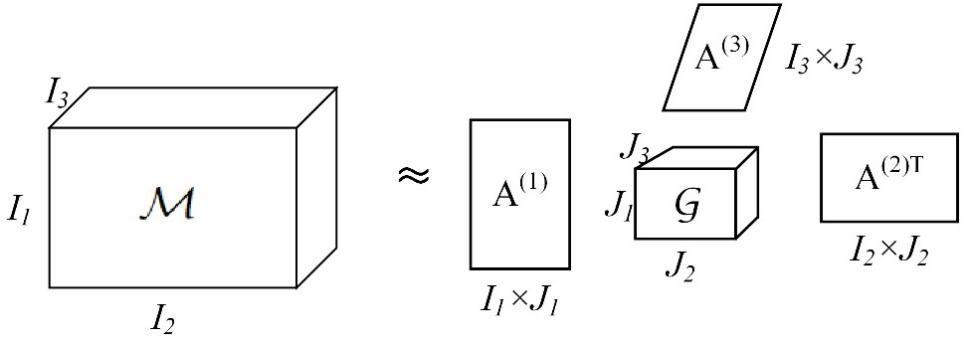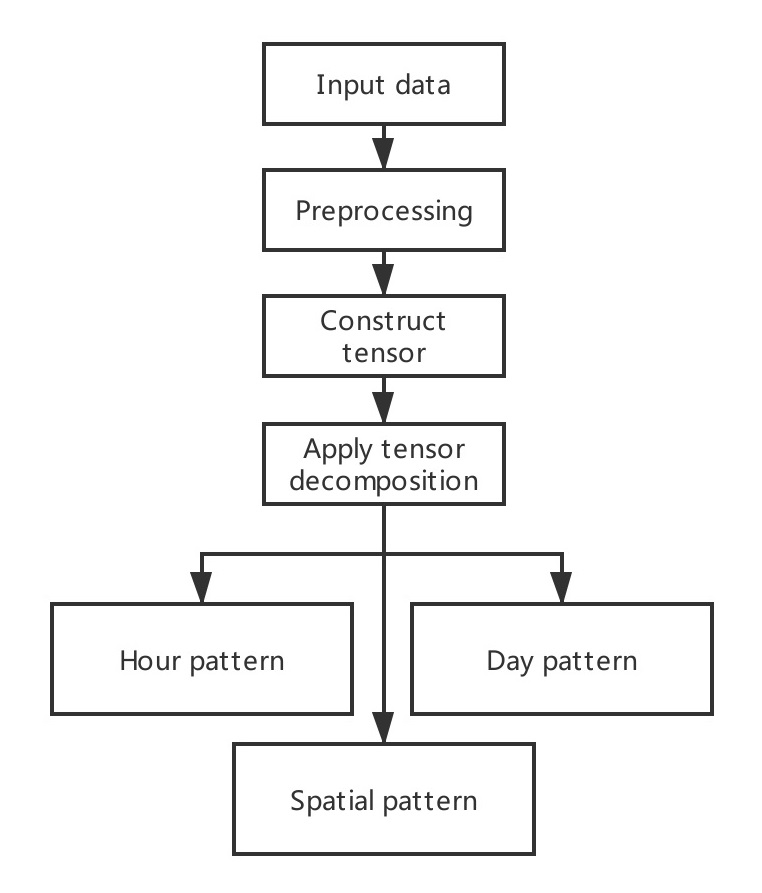## Tensor Decomposition Model on Sharing BicyclesA tensor is a multidimensional array that can be seen as an extension of a matrix in high dimensions (Kolda et al. 2009). In this research, the Tucker decomposition model under nonnegative constraints is used to process the third-order origin and destination tensors of shared bicycles.

Sharing BicyclesTensor DecompositionTraffic
160

#### Contributor#### Authorship

Affiliation:

Email:
caomin@njnu.edu.cn
Is authorship not correct? Feed back

#### Classification(s)

Geography SubjectHuman GeographyTraffic Geography

#### Detailed Description

The public bike-sharing (PBS) system has received increasing attention as a potential way to improve the first and last mile connections to other modes of transit and lessen the environmental impact of transportation activities (DeMaio, 2009; Ruch et al., 2014). Recently, an increasing number of cities have deployed bicycle-sharing systems to solve the first/last mile connection problem, generating a large quantity of data. Additionally, commuting regularities are usually linked to the spatial distribution or the transfer routing of bikes. In this theme, we come up with two models based on mathematical theory aiming to recognize the patterns in sharing bicycle data.
• Tensor Decomposition
A tensor is a multidimensional array that can be seen as an extension of a matrix in high dimensions (Kolda et al. 2009). In this research, the Tucker decomposition model under nonnegative constraints is used to process the third-order origin and destination tensors of shared bicycles.
The purpose of the Tucker decomposition model is to decompose n-dimensional tensor`M∈RI1xI2x...xIn`into a n-dimensional core tensor`G∈RJ1xJ2x...xJn`. Factor matrices can be thought of as the principal components in each dimension of the tensor, and core tensor shows the level of interaction between the different components (Khoromskij et al. 2007). Taking a three-dimensional tensor as an example, the schematic diagram of Tucker decomposition model is shown in Figure 1. The three-dimensional tensor`M∈RI1xI2xI3`is decomposed into a core tensor`G∈RJ1xJ2xJ3`and three factor matrices`A(1)∈RI1xJ1`,`A(2)∈RI2xJ2`,`A(3)∈RI3xJ3`. The formula of Tucker decomposition is shown in equation (1).Figure 1. Illustration of the Tucker decomposition model (Cichocki et al. 2015)

`M≅G x 1A(1) x 2A(2) x 3A(3)`················(1)
Where is a three-dimensional tensor; is a three-dimensional core tensor;`A(1)`,`A(2)` and`A(3)`are three factor matrices.

Each dimension represents a sort of pattern, three patterns can be found after tensor decomposition. The following figure (Figure 2) is the flow chart of Tensor Decomposition.Figure 2. Flow chart of Tensor Decomposition

#### Reference:

DeMaio, P. (2009). Bike-sharing: History, impacts, models of provision, and future. Journal of public transportation, 12(4), 3.

Banerjee, S., & Roy, A. (2014). Linear algebra and matrix analysis for statistics. New York: Chapman and Hall/CRC.

Kolda, T. G., and Bader, B. W. 2009. Tensor decompositions and applications. SIAM review, 51(3), 455-500.

Khoromskij, B., and Khoromskaia, V. 2007. Low rank Tucker-type tensor approximation to classical potentials. Open Mathematics, 5(3), 523-550.

#### Comment(s)

You can link related `{{typeName}}` from your personal space to this model item, or you can create a new {{typeName.toLowerCase()}}.